Teacher: Peter
Customers Who Have Viewed This Course: 1884
\$249.00

#### 0 Course Introduction 06:19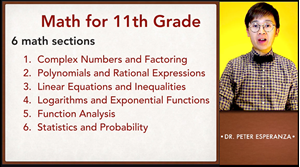This video shows you tips on how you can effectively use the video lessons in learning math for 11th grade.

#### 1 Lesson 1 - Complex Numbers 24:20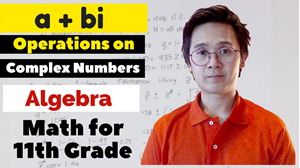This lesson shows you how to perform operations on complex numbers and how to simplify square roots of negative numbers

#### 2 Lesson 1 - Worksheet Review (odd) 08:04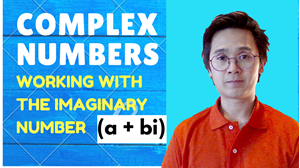This lesson shows you how to answer the odd numbered problems from the worksheet.

#### 3 Lesson 1 - Worksheet Review (even) 07:08

This video shows you how to answer the even number problems from the worksheet.

#### 4 Lesson 2 - Special Role of Zero in Factoring 18:03This lesson shows you how to use the zero product property in solving for the roots or zeros of a polynomial in its factored form.

#### 5 Lesson 2 - Worksheet Review (odd) 07:48this lesson shows you how to answer the odd numbered problems on finding roots of a polynomial.

#### 6 Lesson 2 - Worksheet Review (even) 05:34

This video shows you how to answer the even numbered problems from the worksheet

#### 7 Lesson 3 - Mastering Factoring 29:31this lesson shows you the factoring techniques you need in answering problems involving solutions of polynomial functions.

#### 8 Lesson 3 - Worksheet Review (odd) 08:46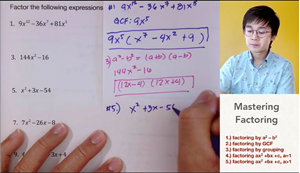This lesson shows you how to answer the odd numbered problems on how to factor polynomials using different techniques.

#### 9 Lesson 3 - Worksheet Review (even) 05:20

This video shows you how to answer the even numbered problems from the worksheet.

#### 10 Lesson 4 - Solving Radical Equations 12:43This lesson shows you how to solve radical equations by taking the power of the roots.

#### 11 Lesson 4 - Worksheet Review (odd) 14:26This video shows you how to answer the odd numbered problems from the worksheet.

#### 12 Lesson 4 - Worksheet Review (even) 09:57

This video shows you how to answer the even numbered problems from the worksheet.

#### 13 Lesson 5 - Properties of Exponents and Radicals 15:31This video shows you how to use the properties of radicals and the law of exponents in solving radical expressions.

#### 14 Lesson 5 - Worksheet Review (odd) 07:51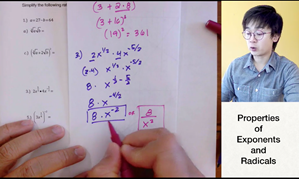This lesson shows you how to answer the odd numbered problems from the worksheet.

#### 15 Lesson 5 - Worksheet Review (even) 09:46

This video shows you how to answer the even numbered problems from the worksheet.

#### 16 Lesson 6 - Arithmetic Operations on Polynomials 20:36This lesson shows you how to add, subtract, multiply, and divide polynomials.

#### 17 Lesson 6 - Worksheet Review Part 1 (odd) 10:23This lesson will show you how to answer the ODD numbered problems from the worksheet. This video is on practicing on how to change a polynomial in its standard form. Standardizing polynomials sometimes require you to add, subtract, multiply and divide polynomials.

#### 18 Lesson 6 - Worksheet Review Part 2 (division) 12:14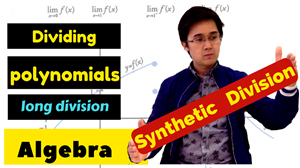This lesson shows you how to divide polynomials using long division and synthetic division. This video will help you in answering problems involving division of polynomials with a binomial as a divisor.

#### 19 Lesson 6 - Worksheet Review Part 3 (even) 06:28

This video shows you how to answer the even numbered problems from the worksheet.

#### 20 Lesson 7 - Remainder & Factor Root Theorems 16:29This lesson shows you how to find the roots of polynomials using the Factor Root Theorem and Remainder Theorem. In this method, synthetic division will be used to verify possible solutions or roots of polynomials.

#### 21 Lesson 7 - Worksheet Review (odd) 14:36This lesson shows you how to use the Remainder Theorem and Factor Root Theorem in solving non factorable polynomials.

#### 22 Lesson 7 - Worksheet Review (even) 10:15

This video shows you how to answer the even numbered problems from the worksheet.

#### 23 Lesson 8 - Simplifying Rational Expressions 10:49This lesson shows you how to use the Law of Exponents and factoring method in simplifying rational expressions.

#### 24 Lesson 8 - Worksheet Review (odd) 05:49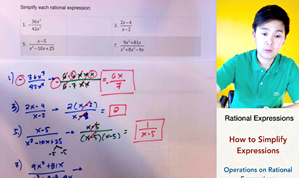this lesson show you how to answer the odd numbered problem from the worksheet.

#### 25 Lesson 8 - Worksheet Review (even) 05:14

This video shows you how to answer even numbered problems on simplifying rational expressions

#### 26 Lesson 9 - Multiplying and Dividing Rational Expressions 12:38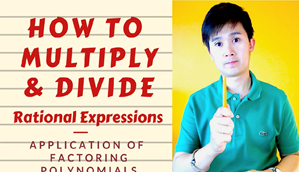This lesson shows you how to multiply and divide rational expressions. Multiplication and division of rational expression is similar to how you multiply and divide regular fractions. You need to use the Law of Exponents and factoring technique in simplifying rational expressions.

#### 27 Lesson 9 - Worksheet Review (odd) 07:20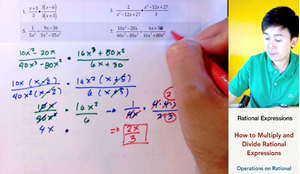this lesson shows you how to answer the odd numbered problem on multiplying and dividing rational expressions from the worksheet.

#### 28 Lesson 9 - Worksheet Review (even) 06:03

This video shows you how to answer the even numbered problems from the worksheet.

#### 29 Lesson 10 - Adding and Subtracting Rational Expressions 04:32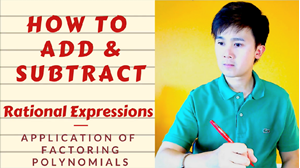This lesson shows you how to add and subtract rational expressions. The process of performing this operation is similar to adding and subtracting regular fractions.

#### 30 Lesson 10 - Worksheet Review (odd) 07:04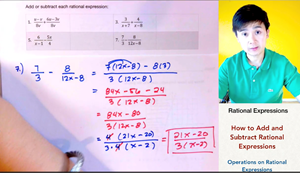This lesson shows you how to add and subtract rational expressions.

#### 31 Lesson 10 - Worksheet Review (even) 07:14

This video shows you how to answer the even numbered problems from the worksheet.

#### 32 Lesson 11 - Square Root of Positive Radical Expressions 08:20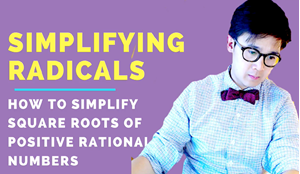This lesson shows you how to simplify algebraic expressions in a square root.

#### 33 Lesson 11 - Worksheet Review (odd) 09:28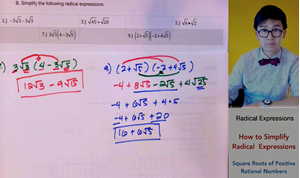This lesson shows you how to simplify radical expressions and evaluate expressions in a square root.

#### 34 Lesson 11 - Worksheet Review 07:45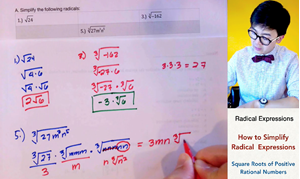This video shows you how to answer the even numbered problems from the worksheet.

#### 35 Lesson 12 - Solving Systems of Linear Inequalities 09:05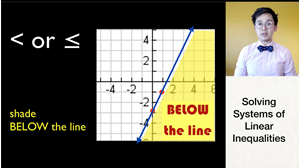This lesson shows you how to shade the region of the solution set of the systems of linear inequalities.

#### 36 Lesson 12 - Worksheet Review 12:39This lesson shows you how to find the solution set of a system of linear inequalities by graphing.

#### 37 Lesson 13 - Solving Systems by Graphing 06:46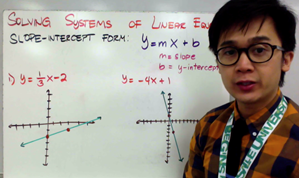This lesson shows you how to solve systems of linear equations using graphing method. To better understand this lesson, you should know how to graph a linear equation in the slope-intercept form (y=mx+b).

#### 38 Lesson 13 - Worksheet Review (odd) 10:53This lesson contains solution video on ODD numbered problem from the WORKSHEET.

#### 39 Lesson 13 - Worksheet Review (even) 07:08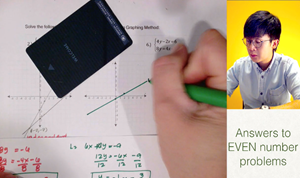This video shows you how to answer the even numbered problems from the worksheet.

#### 40 Lesson 14 - Solving Systems Using Substitution Method 09:01

This lesson show you how to solve systems of linear equations using the substitution method. In this method, you will solve the system algebraically.

#### 41 Lesson 14 - Worksheet Review (odd) 09:11This lesson contains solution video on ODD numbered problem from the WORKSHEET.

#### 42 Lesson 14 - Worksheet Review (even) 04:42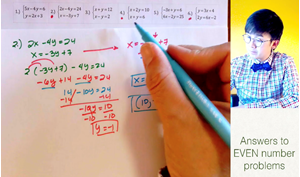This video shows you how to answer the even number problems on solving linear system by substitution

#### 43 Lesson 15 - Solving Systems by Elimination Method 09:39

This lesson shows you how to solve systems of linear equations using the ELIMINATION method. In this method, you will solve the system algebraically by finding "zero pairs" in the system.

#### 44 Lesson 15 - Worksheet Review (odd) 07:47This lesson contains solution video on ODD numbered problem from the WORKSHEET.

#### 45 Lesson 15 - Worksheet Review (even) 04:33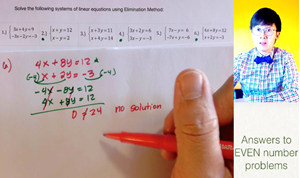This video shows you how to solve problems on finding the solutions of linear system by substitution.

#### 46 Lesson 16 - Finding the Determinants of a Linear System 06:15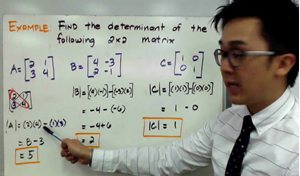This lesson is your prerequisite in learning how to find the solutions of a linear system with 3 VARIABLES (x,y,z). In this lesson, you will learn how to find the determinant of a 2x2 and 3x3 matrix which will help you in finding the solutions of a 3-variable linear system.

#### 47 Lesson 16 - Worksheet Review (odd) 08:27This lesson shows you how to find the determinant of a 2x2 and a 3x3 matrix which is a prerequisite in solving linear system using linear algebra

#### 48 Lesson 16 - Worksheet Review (even) 05:11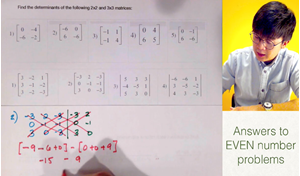This video shows you how to answer the even numbered problems from the worksheet.

#### 49 Lesson 17 - Solving Systems with 3 Variables by Cramer's Rule 08:03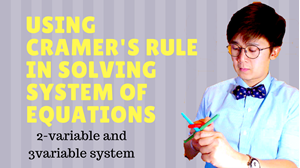This lesson will show you a more systematic way on how to solve linear system with 3 variables (x,y,z). In using Cramer's rule, you will need to convert your linear system into an augmented matrix to solve the system. Finding the determinant of each matrix is your key in solving for the solution of this 3-variable system of equations.

#### 50 Lesson 17 - Worksheet Review (odd) 14:59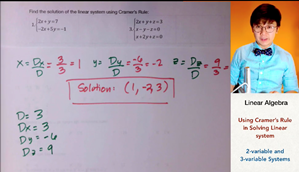This lesson shows you how to use the determinants of a matrix to find the solutions of a linear system with 2 variables and 3 variables. Cramer's rule is used to solve system when you convert your linear system to its augmented matrix.

#### 51 Lesson 17 - Worksheet Review (even) 09:26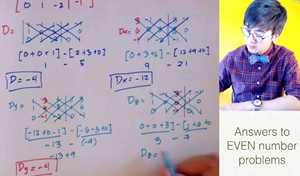This video shows you how to answer the even numbered problems from the worksheet.

#### 52 Lesson 18 - Factoring Quadratic Equations 18:51

This lesson shows you how to solve quadratic equations and find its solutions using factoring method.

#### 53 Lesson 18 - Worksheet Review (odd) 07:16This lesson shows you how to answer the odd numbered problems on factoring quadratic equations.

#### 54 Lesson 18 - Worksheet Review (even) 05:13

This video shows you how to answer the even numbered problems from the worksheet.

#### 55 Lesson 19 - Solving Special Cases of Quadratic Equations 19:01

This lesson shows you 2 methods on how to find the solutions of quadratic equations.

#### 56 Lesson 19 - Worksheet Review 08:08This lesson shows you how to answer the problems on finding the solutions of quadratic equations using different methods from the worksheet.

#### 57 Lesson 20 - Solving Non-Factorable Quadratic Equations 20:10

This lesson shows you how to solve NON-FACTORABLE quadratics using the quadratic formula and completing the square techniques in finding the solutions of a quadratic equation

#### 58 Lesson 20 - Worksheet Review 18:04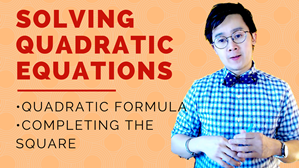This lesson shows you how to answer the problems on solving NON-FACTORABLE quadratic equations.

#### 59 Lesson 21 - Evaluating Logarithmic Expressions 07:24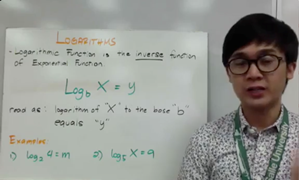This lesson shows you how to evaluate logarithmic expressions by converting logarithms to exponential equations.

#### 60 Lesson 21 - Worksheet Review Part 1 06:26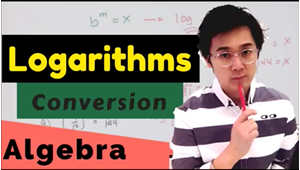This video shows you how to answer problems on converting logarithms.

#### 61 Lesson 21 - Worksheet Review Part 2 11:38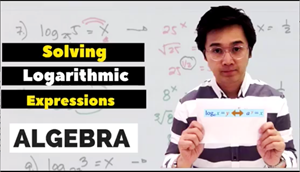This video shows you how to evaluate logarithmic expressions.

#### 62 Lesson 22 - Properties of Logarithms 14:36

This video shows you how to use the properties of logarithms in simplifying logarithmic expressions and equations.

#### 63 Lesson 22 - Worksheet Review 09:02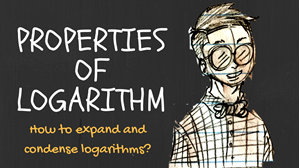This video shows you how to answer the odd numbered problems on simplifying logarithms using its properties.

#### 64 Lesson 23 - Solving Logarithmic Equations 06:23

This lesson shows you how to solve logarithmic equations using the properties of logarithms.

#### 65 Lesson 23 - Worksheet Review 10:48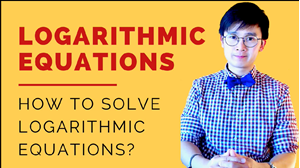This video shows you how to answer the problems on how to solve logarithmic equations.

#### 66 Lesson 24 - Graph and Solutions of Exponential Functions 16:08This lesson shows you how to solve and graph exponential functions by applying the properties of logarithms. It also shows you the transformations of an exponential functions.

#### 67 Lesson 24 - Worksheet Review 07:58This video shows you how to solve the odd numbered problems on how to solve exponential functions and its transformations.

#### 68 Lesson 25 - Transformations of the Graphs of Logarithmic Functions 10:06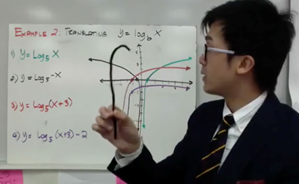This lesson shows you how to graph logarithmic equations and its translations in the x-y plane.

#### 69 Lesson 25 - Worksheet Review 10:02This lesson shows you how to graph logarithmic functions and its transformations.

#### 70 Lesson 26 - Modeling a Function 12:07

This lesson shows you how to model functions to check the behavior of specific functions with different degrees.

#### 71 Lesson 26 - Worksheet Review 14:39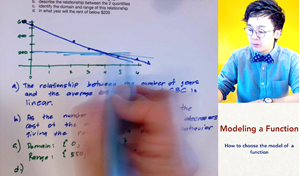This video shows you how to solve modeling problems involving functions from the worksheet.

#### 72 Lesson 27 - Function Arithmetic Operations 08:21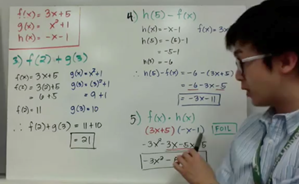This lesson shows you how to use some arithmetic operations Involving functions.

#### 73 Lesson 27 - Worksheet Review 04:09This lessons shows you how to answer the problems involving arithmetic operations of functions.

#### 74 Lesson 28 - Composite Functions 08:24This video shows you how to perform composition of functions.

#### 75 Lesson 28 - Worksheet Review 06:34This video shows you how to answer problems on composite functions.

#### 76 Lesson 29 - Solving and Graphing Inverse of a Function 08:53This video shows you how to solve and graph the inverse of a function.

#### 77 Lesson 29 - Worksheet Review 12:16This video shows you how to answer the problems involving inverse of a function.

#### 78 Lesson 30 - Trigonometric Functions 17:06This lesson shows you how to graph sine and cosine functions and its inverses.

#### 79 Lesson 30 - Worksheet Review 16:45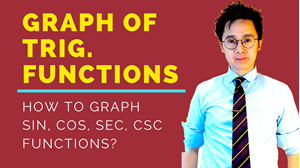This video shows you how to graph the sine and cosine functions as well as its inverses.

#### 80 Lesson 31 - Classical Probability 09:43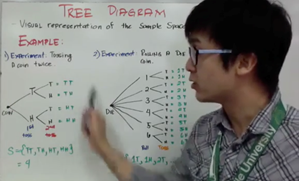This lesson shows you how to compute for the probability of an event given its sample space.

#### 81 Lesson 31 - Worksheet Review 08:15This lesson shows you how to solve the problems on classical probability from the worksheet.

#### 82 Lesson 32 - Conditional Probability 10:35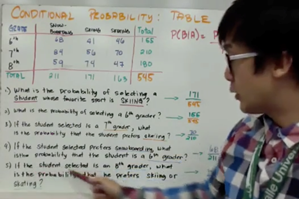This lesson shows you how to compute for the conditional probability and events given a 2-way table.

#### 83 Lesson 32 - Worksheet Review 09:00This lesson shows you how to compute for the conditional probability from the worksheet.

#### 84 Lesson 33 - Probability of Events Given a Venn DIagram 15:41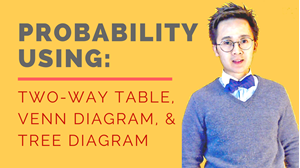This lesson on probability shows you how to compute for the probability of an event given its Venn diagram.

#### 85 Lesson 33 - Worksheet Review 15:43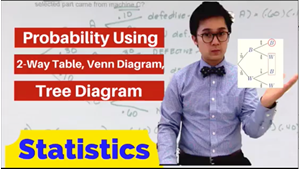This video shows yo how to answer probabilities of an event given its Venn diagram.

#### 86 Lesson 34 - Probability Rules 07:42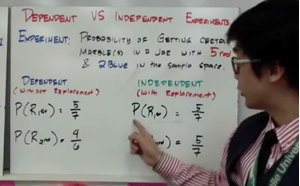This lesson shows you how to answer probability of dependent and independent events.

#### 87 Lesson 34 - Worksheet Review 12:28This video shows you how to answer problems involving the probability rules of dependent and independent events.

#### 88 Lesson 35 - Measure of Center, Shape, Spread 10:59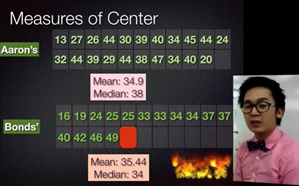This lesson shows you the measures of the center, shape, and spread of a distribution.

#### 89 Lesson 35 - Worksheet Review 08:35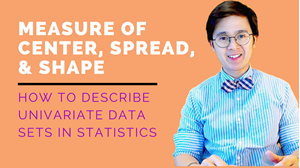This lesson shows you how to describe the shape, spread, and center of a distribution.

#### 90 Lesson 36 - Normal Distribution 68-95-99.7 05:08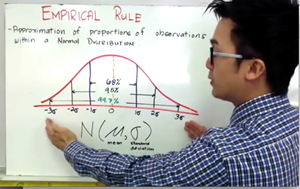This lesson shows you how to estimate the area under the Normal distribution using the empirical rule.

#### 91 Lesson 36 - Worksheet Review 11:48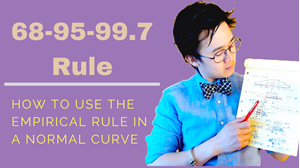This lesson shows you how to compute for the proportion or percentage using the Normal curve.

#### 92 Lesson 37 - Computing for Percentiles on a Normal Curve 07:46This lesson shows you how to compute for the percentile of a Normal distribution using the Z-score.

#### 93 Lesson 37 - Worksheet Review 18:20This shows you how to answer the statistics problem on percentile in the worksheet.

#### 94 Lesson 38 - Computing for Normal Probability 09:36

This lesson shows you how to answer problems involving Normal probability.

#### 95 Lesson 38 - Worksheet Review 16:50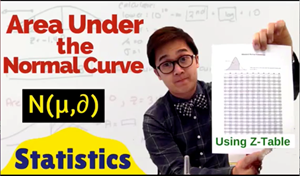This lesson shows you how to compute for Normal probability.

#### 96 Lesson 39 - Population and Sample 09:21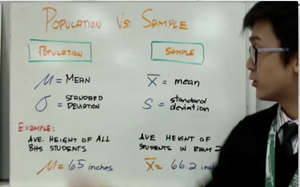This lesson shows you the difference between population and sample in statistics.

#### 97 Lesson 39 - Worksheet Review 06:04This lesson shows you how to answer problems involving the difference between population and sample

Course Description

Math for 11th grade builds your intermediate algebra skill on working with linear, quadratic, and exponential functions.  The course extends on the topics of functions which include polynomial, rational, radical, logarithmic, exponential, and trigonometric functions. You will also work closely with the expressions that define the functions, and continue to expand and hone your abilities to model situations and to solve equations, including solving quadratic equations over the set of complex numbers and solving exponential equations using the properties of logarithms.

Course Goals

Upon completion of this course, students will be prepared to move on to math courses which demands strong algebra skills such as: calculus, statistics, trigonometry, analytic geometry, precalculus, linear algebra, and some college courses like calculus 1, calculus 2, and introductory statistics.

Course Requirements

Student taking this course are expected to have basic algebra skills such as solving linear equation, factoring quadratics, and simplifying rational expressions.

Target Audience

This video course is primarily intended for students who are interested in taking higher math elective classes at the high school and math classes in college. This course will also help students in building their mathematical and analytical skills which will prepare them in taking other courses in mathematics and the sciences.

Technology

Some of the math topics covered in this course use Ti-84 or Ti-83 in solving math problems.  The use of any mobile apps like Desmos can also be helpful in understanding behavior of functions through its graph.

Course Includes

•  18 hours of video

97 in depth video lessons (I slowly explain each new concept)

•  58 worksheets

58 worksheet review videos (I explain each answer step by step)

Over 400 exercises

•  Answer keys to all tests (at the bottom of the worksheet)

Suggested routine in learning math for 11th grade curriculum:

Step 1) Watch the lesson video.

Step 2) Copy the lesson video in your notebook

Step 3) Watch the Worksheet Review Video to compare your answers using the teacher’s worked out math solutions.

SECTION 1: COMPLEX NUMBERS AND FACTORING

LESSON 1 -  Complex numbers

LESSON 2 -  Special role of zero in factoring

LESSON 3 - Mastering Factoring

LESSON 4 - Solving Radical Equations

LESSON 5 - Properties of Exponents and Radicals

SECTION 2: POLYNOMIALS, RADICALS, AND RATIONALS

LESSON 6 - Arithmetic operations on polynomials

LESSON 7 - Remainder and factor root theorems

LESSON 8 - Simplifying Rational Expressions

LESSON 9 - Multiplying and dividing rational expressions

LESSON 10 - Adding and subtracting rational expressions

LESSON 11 - Square root of positive radical expressions

SECTION 3: SYSTEMS OF LINEAR EQUATIONS AND INEQUALITIES

LESSON 12 - Solving systems of linear inequalities

LESSON 13 - Solving systems using graphing method

LESSON 14 - Solving systems using substitution method

LESSON 15 - Solving systems using elimination method

LESSON 16 - Finding the determinants of a linear system

LESSON 17 - Solving systems of equations using Cramer’s Rule

SECTION 4: EXPONENTIAL AND LOGARITHMIC FUNCTIONS

LESSON 18 - Factoring quadratic equations

LESSON 19 - Solving special cases of quadratic equations

LESSON 20 - Solving non-factorable quadratic equations

LESSON 21 - Evaluating logarithmic expressions

LESSON 22 - Properties of logarithms

LESSON 23 - Solving logarithmic equations

LESSON 24 - Graph and solutions of exponential functions

LESSON 25 - Transformations of the graphs of logarithmic functions

SECTION 5: FUNCTION OPERATIONS AND ANALYSIS

LESSON 26 - Modeling a function

LESSON 27 - Function arithmetic operations

LESSON 28 - Composite Functions

LESSON 29 - Solving and graphing inverse of a function

LESSON 30 - Graphing trigonometric functions

SECTION 6: STATISTICS AND PROBABILITIES

LESSON 31 - Classical probability

LESSON 32 - Conditional probability

LESSON 33 - Probability of events given a venn diagram

LESSON 34 - Using probability rules

LESSON 35 - Measure of center, shape, and spread.

LESSON 36 - Using Normal distribution: 68-95-99.7 percent rule

LESSON 37 -  Computing for percentile of a Normal curve

LESSON 38 - Computing Normal probability

LESSON 39 - Differentiating populations and sample.

• Teacher: Peter
• Areas of expertise: Mathematics
• Education: EdD-Development Education • MAT-Math • BS-Mathematics • BSEd-Computer Applications
• Interests: Crossfit, Tough mudder, web development, and cooking.
• Skills: I can do handstand push-ups and double unders.
• Associations: Apple Distinguished Educator, National Council of Teachers of Mathematics, and California Teachers Association